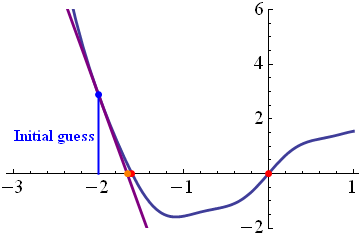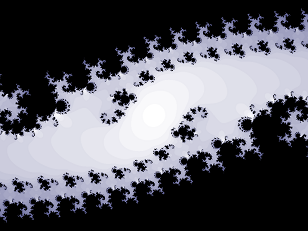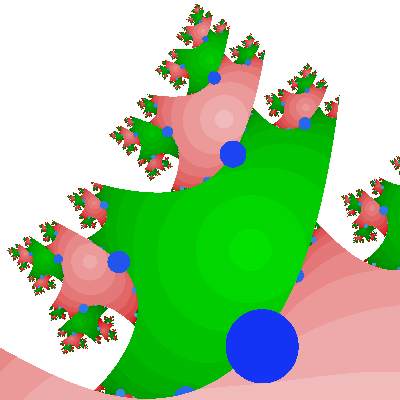## Monday, November 10, 2008

### Fractals from Newton's Method

Today, I will explain how I created this:This is a fractal. A fractal is a pattern that contains smaller versions of itself. But it's not just any fractal. It's a fractal I created from something called Newton's method.

Newton's Method

Let's say we have a mathematical function called f(x). I chose one specifically for this demonstration. Here is a graph:A very common math problem is to find the "roots" of f(x). That means you're trying to find what numbers "x" can you use to make f(x) equal to zero. In a graph, that means that it touches the horizontal axis. In the picture above, the roots are all shown with red dots. You can see that one root is zero, and the others are near 2 and -2.

It's easy for me to make an instant estimate of the roots, but that's because I had a computer graph it for me. What if I were, say, Isaac Newton, and I had no computers? What if I wanted a really accurate estimate of the roots? I would invent a new mathematical method and name it after myself, of course. And that's what Newton did.

Newton's method relies on the fact that most functions are more or less straight. The graph of f(x) sure doesn't look straight--it curves all over the place. But if we zoomed on just one part of the graph, it would be almost straight. An almost-straight line is almost like a straight line. So it stands to reason that an almost-straight line has almost the same root as a straight line.In the above graph, I started by "guessing" the location of the root at -2. Using this guess, I drew a "tangent line" to f(x). This tangent line is a straight line that just barely touches f(x) at the blue point. Finding a tangent line is a standard method from calculus. If we just find the root of the tangent line, we know it must be fairly close to the root of f(x).

Notice that we started with an initial guess of -2, and we got a much better guess. That means we can take any guess and make it into a better guess! There's no reason to stop there. All we need to do is repeat the process, starting with a better guess each time. You can get a very good estimate of the root of f(x) very quickly.

The trouble with Newton's method is that functions aren't really straight. They can curve all over the place! Let's see what happens when I try a different initial guess of -1.After only one iterations, it looks like we're getting a very accurate approximation of the root near 2. But wait, didn't we initially guess -1? Even though our initial guess is between the first two roots, we end up finding the third root. Newton would probably consider this a bad guess, because we didn't find the root we wanted to. However, we have a different idea in mind.

We want to answer the question: Given any initial guess, which root will we eventually find?

Though the original method was invented in the time of Newton, this is a question that they never could have answered. What if a single guess bounces around for a while, before finding a root? You really need to use a computer to test all the possibilities. So that's what I did.(Click for a bigger picture.) When you guess badly, you get a fractal!

Allow me to explain the meaning of the fractal. Each color corresponds to a different root. The darkness of the color corresponds to the number of iterations required to get the root. Of course, you never quite reach the root exactly. But once it's within a certain distance, the computer decides that it's close enough. Some guesses are so bad that they don't ever find any root (at least as far as my computer has tried). Those guesses are indicated in white.

It's actually not too surprising that this method would result in fractals. First you have the large regions which correspond to good guesses. Then you have small regions of bad guesses. These "bad guess" regions map to the rest of the number line. And so, the "bad guess" regions will end up looking like smaller versions of the entire fractal.

More complex, More fractal

So far, I've only explained how to make a 1-dimensional fractal. The one-dimensional fractal maps the number line to different colors. But at the top, I showed you a 2-dimensional fractal. The 2-dimensional fractal maps the complex plane to different colors.

The complex plane is a sort of extension of the number line into two dimensions. It includes the "real" numbers, like -1, pi, and sqrt(2). It also includes "imaginary" numbers, like "i", the square root of negative one. And then there are complex numbers, which are in the form a+b*i. The number "a" is called the real part, and "b" is called the imaginary part. The real part is represented by the horizontal position on the complex plane, while the imaginary part is represented by the vertical position.

Otherwise, the method is exactly the same. Only now, it's prettier. And there might be new roots that were previously hidden.

The fractal at the top was generated using the function f(x) = x^3-1

But I have tried much more complicated functions as well. Some of you might recognize this one, because I use it as my avatar in certain internet locales.This one was generated by the function f(x) = x*cos(x)^i. This function has only one root. The black regions correspond to guesses that never lead to the root.This was generated by the function f(x) = log(x) + x. I also made a nice desktop-sized version, 'cause it's so awesome.This is the function f(x) = ex - x. This function has an infinite number of roots, only two of which are being shown.This is f(x) = log(x2). I had posted this on my blog last Christmas. The blue "ornaments" are actually an exploit in my computer program; they wouldn't normally be there.

These are all generated using a Java program that I made for a high school project. I have found it very fun to experiment with this math-to-art device. I want you all to have a taste of that. So... later, I will be taking requests for mathematical functions!Anonymous said...

Very nice--both the explanation and the fractals themselves! I love how many different questions can lead to fractals as answers--which reminds me that I need to write a program to generate the Mandelbrot set before I write my next rant…Anonymous said...

Oops, I forgot to ask: how are you choosing colors based on the roots that are found? Why are you getting a gradient of color in some areas?

miller said...

The color depends on two things: which root it converges on, and how many iterations it required to get within a certain range (it's assumed to converge after that). So for example, in the wave-shaped fractal, if you find the first root within a few iterations, it will appear orange. But the more iterations it takes, the more reddish it will appear. If you find the second root within a few iterations, it will appear blue, but if it takes more iterations, it will appear cyan.

The specific colors, orange and red, blue and cyan, are chosen by me for their aesthetic value.

Kaz Maslanka said...

Very nice explanation
Thanks!
Kaz

NiteSkyGirl said...

intelligent !!
enjoyed reading this - did 4 times.